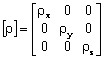# Isotropic / anisotropic materials

## Introduction

The studied materials can be isotropic or anisotropic. In other words, the magnetic behavior of the material is as follows:

• independent of the direction of the applied field (isotropic material)
• dependent on the direction of the applied field (anisotropic material)

These two cases are presented in the following sections.

## Isotropic materials

Isotropic materials are characterized by a magnetization law independent of the direction of the applied field.

The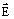and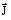vectors are always collinear.

The dependence betweenandis a scalar relationship, which is written as: J= σ.E or E= ρ.J

## Anisotropic materials

Anisotropic materials are characterized by a law, which is dependent on the direction of the applied field.

Theandvectors are not collinear.

The dependence betweenandis a vector relationship, which is written as: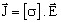or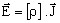with σ conductivity tensor: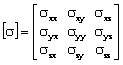or ρ resistivity tensor: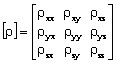## … in Flux

The model provided in Flux is a simplified model.

The vector dependence betweenandwhich is written as:orcan therefore be expressed:

• either under the form of three curves: Jx(Ex), Jy(Ey), Jz(Ez)

The conductivity tensor is then written: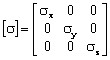• or under the form of three curves: Ex(Jx), Ey(Jy), Ez(Jz)

The resistivity tensor is then written: